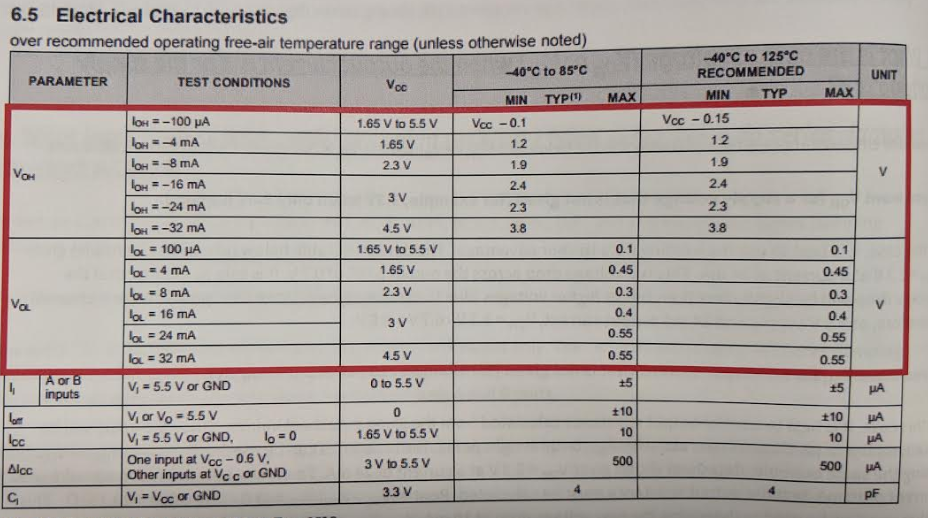If you have a related question, please click the "Ask a related question" button in the top right corner. The newly created question will be automatically linked to this question.

# [FAQ] What is the output voltage (VOH or VOL) when the output current is X or the supply voltage is Y?

FAQ: Logic and Voltage Translation > Output Parameters >> Current FAQ

There are circumstances where you might want to know a VOH or VOL Value that is not given. I will describe two cases:

If you want VOH for a supply voltage that is not given (for example, 3.3V when only 3.0V is given):
In this case, it is best to use the existing data to your advantage The datasheet table below (used as an example) gives VOH = 2.3 V at a current of 24 mA. This is a voltage drop across the output PFET of 0.7V. It is safe to assume that the voltage drop will be slightly less than this at higher voltages (due to decreasing resistance as supply voltage increases). Therefore, at 3.3 V supply and 24 mA output current, VOH = 3.3 V - 0.7V = 2.6V

If you want VOH for an output current that is not given (for example, 10 mA at 3.0 V supply):
In this case, it is best to use the output resistance calculated from the given datasheet values. Since the value will be relatively linear, the resistance can be used to calculate VOH or VOL. Using the same example, datasheet shows gives VOH = 2.3 V at a current of 24 mA. To get the maximum output voltage at a current of 10 mA, first the output resistance must be calculated: Rout= (Vcc-VOH)/IOH = (3.0 - 2.3)/0.024 = 29.167Ohms. This value can then be used to determine the new voltage drop at 10 mA: Vcc-VOH = 0.01 * Rout = 0.29167 V. Solving for VOH yields: VOH = Vcc - 0.29167 ~= 2.71 V

*** What follows is a more detailed explanation of the above ***
A CMOS device's output voltage is primarily determined by the output driver's impedance and the amount of current it is sourcing or sinking. This impedance changes depending on Vcc, so each device's datasheet will include VOH and VOL values across multiple supply values, and each will include a current under the test conditions. One example is shown here:In the Electrical Characteristics table, there are typically values given for low current (100 uA) and maximum recommended current at each supply range.

The voltage drop across the output MOSFET's is essentially what is being described here.It is important to note that the output resistance for a CMOS driver changes across supply and output current. Given that the supply voltage is held constant, the output resistance is relatively linear over the typical operating range. In the above image, you can see that the output current rating and output voltage rating fall very close to an idealized constant resistance line. Knowing this simple fact will make it relatively easy to estimate the output voltage and current under many scenarios. The datasheet given values can be used to calculate the output resistance at a given supply value, then other values can be obtained using simple circuit analysis techniques.